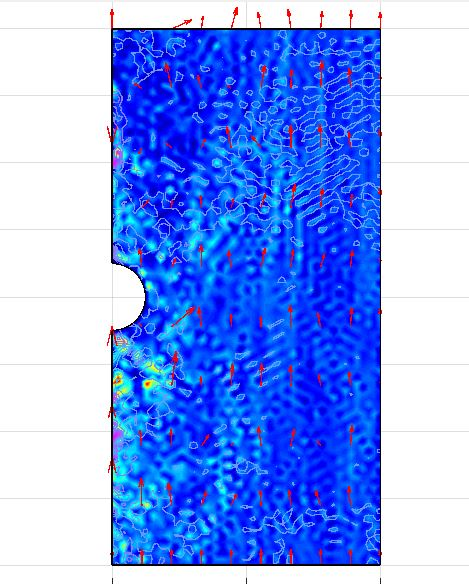# how to calculate the drag force of a sphere in axisymmetryClassicListThreaded3 messagesOpen this post in threaded view
|

## how to calculate the drag force of a sphere in axisymmetry

 Hi I have a rather basic simulation that I would like to preform that can be compared to reference values. What I want to do is to simulate the drag force of a sphere in axisymmetry setup. I have manage build the model and run the simulation and the velocity plot looks reasonable. My question is how I should define the correct equation for drag force in the Boundary Integration box (for axisymmetry)? My thanks for help in advance! BRAdministrator Similar to the flow around a cylinder benchmark example https://www.featool.com/doc/fluid_dynamics_04_flow_around_cylinder1you would need to derive and integrate the (axisymmetric) expression for the Total-stress tensor in the z-direction, which should be something like ``` fz = r*(nz*p+miu*(-nr*(wr+uz)-2*nz*wz)) cd = 2*fz/(rho*umean^2*diam) ```That said, the solution in the attached image does not look converged as fluctuates a lot between grid cells. Depending on the flow regime you might need to increase the mesh density, use artificial (numerical) stabilization, and/or a turbulence model.